Contents | Prev | Next | Index

# 20.11 The Class `java.lang.Math`

The class `Math` contains useful basic numerical constants and methods.

```public final class `Math` {
public static final double `E` = 2.7182818284590452354;
public static final double `PI` = 3.14159265358979323846;
public static double `sin`(double a);
public static double `cos`(double a);
public static double `tan`(double a);
public static double `asin`(double a);
public static double `acos`(double a);
public static double `atan`(double a);
public static double `atan2`(double a, double b);
public static double `exp`(double a);
public static double `log`(double a);
public static double `sqrt`(double a);
public static double `pow`(double a, double b);
public static double `IEEEremainder`(double f1, double f2);
public static double `ceil`(double a);
public static double `floor`(double a);
public static double `rint`(double a);
public static int `round`(float a);
public static long `round`(double a);
public static double `random`();
public static int `abs`(int a);
public static long `abs`(long a);
public static float `abs`(float a);
public static double `abs`(double a);
public static int `min`(int a, int b);
public static long `min`(long a, long b);
public static float `min`(float a, float b);
public static double `min`(double a, double b);
public static int `max`(int a, int b);
public static long `max`(long a, long b);
public static float `max`(float a, float b);
public static double `max`(double a, double b);
}
```
To ensure portability of Java programs, the specifications of many of the numerical functions in this package require that they produce the same results as certain published algorithms. These algorithms are available from the well-known network library `netlib` as the package `fdlibm` ("Freely Distributable Math Library"). These algorithms, which are written in the C programming language, are to be understood as if executed in Java execution order with all floating-point operations following the rules of Java floating-point arithmetic.

The network library may be found at `http://netlib.att.com` on the World Wide Web; then perform a keyword search for `fdlibm`. The library may also be retrieved by E-mail; to begin the process, send a message containing the line:

```send index from fdlibm
```
to `[email protected]`. The Java math library is defined with respect to the version of `fdlibm` dated 95/01/04. Where `fdlibm` provides more than one definition for a function (such as `acos`), the "IEEE754 core function" version is to be used (residing in a file whose name begins with the letter `e`).

A complete and self-contained description of the algorithms to be used for these functions will be provided in a future version of this specification. It is also anticipated that the algorithms will be coded in Java to provide a reference implementation that is not tied to `fdlibm`.

20.11.1 `public static final double E = 2.7182818284590452354;`

The constant value of this field is the `double` value that is closer than any other to e, the base of the natural logarithms.

20.11.2 `public static final double PI = 3.14159265358979323846;`

The constant value of this field is the `double` value that is closer than any other to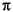, the ratio of the circumference of a circle to its diameter.

20.11.3 `public static double sin(double a)`

This method computes an approximation to the sine of the argument, using the `sin` algorithm as published in `fdlibm` (see the introduction to this section).

Special cases:

• If the argument is NaN or an infinity, then the result is NaN.
• If the argument is positive zero, then the result is positive zero; if the argument is negative zero, then the result is negative zero.

20.11.4 `public static double cos(double a)`

This method computes an approximation to the cosine of the argument, using the `cos` algorithm as published in `fdlibm` (see the introduction to this section).

Special case:

• If the argument is NaN or an infinity, then the result is NaN.

20.11.5 `public static double tan(double a)`

This method computes an approximation to the tangent of the argument, using the `tan` algorithm as published in `fdlibm` (see the introduction to this section).

Special cases:

• If the argument is NaN or an infinity, then the result is NaN.
• If the argument is positive zero, then the result is positive zero; if the argument is negative zero, then the result is negative zero.

20.11.6 `public static double asin(double a)`

This method computes an approximation to the arc sine of the argument, using the a`sin` algorithm as published in `fdlibm` (see the introduction to this section).

Special cases:

• If the argument is NaN or its absolute value is greater than 1, then the result is NaN.
• If the argument is positive zero, then the result is positive zero; if the argument is negative zero, then the result is negative zero.

20.11.7 `public static double acos(double a)`

This method computes an approximation to the arc cosine of the argument, using the `acos` algorithm as published in `fdlibm` (see the introduction to this section).

Special case:

• If the argument is NaN or its absolute value is greater than 1, then the result is NaN.

20.11.8 `public static double atan(double a)`

This method computes an approximation to the arc tangent of the argument, using the a`tan` algorithm as published in `fdlibm` (see the introduction to this section).

Special cases:

• If the argument is NaN, then the result is NaN.
• If the argument is positive zero, then the result is positive zero; if the argument is negative zero, then the result is negative zero.

20.11.9 `public static double atan2(double y, double x)`

This method computes an approximation to the arc tangent of the quotient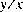of the arguments, using the a`tan2` algorithm as published in `fdlibm` (see the introduction to this section).

Special cases:

• If either argument is NaN, then the result is NaN.
• If the first argument is positive zero and the second argument is positive, or the first argument is positive and finite and the second argument is positive infinity, then the result is positive zero.
• If the first argument is negative zero and the second argument is positive, or the first argument is negative and finite and the second argument is positive infinity, then the result is negative zero.
• If the first argument is positive zero and the second argument is negative, or the first argument is positive and finite and the second argument is negative infinity, then the result is the `double` value closest to.
• If the first argument is negative zero and the second argument is negative, or the first argument is negative and finite and the second argument is negative infinity, then the result is the `double` value closest to.
• If the first argument is positive and the second argument is positive zero or negative zero, or the first argument is positive infinity and the second argument is finite, then the result is the `double` value closest to.
• If the first argument is negative and the second argument is positive zero or negative zero, or the first argument is negative infinity and the second argument is finite, then the result is the `double` value closest to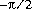.
• If both arguments are positive infinity, then the result is the `double` value closest to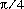.
• If the first argument is positive infinity and the second argument is negative infinity, then the result is the `double` value closest to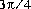.
• If the first argument is negative infinity and the second argument is positive infinity, then the result is the `double` value closest to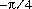.
• If both arguments are negative infinity, then the result is the `double` value closest to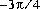.

20.11.10 `public static double exp(double a)`

This method computes an approximation to the exponential function of the argument (e raised to the power of the argument, where e is the base of the natural logarithms (§20.11.1)), using the `exp` algorithm as published in `fdlibm` (see the introduction to this section).

Special cases:

• If the argument is NaN, then the result is NaN.
• If the argument is positive infinity, then the result is positive infinity.
• If the argument is negative infinity, then the result is positive zero.

20.11.11 `public static double log(double a)`

This method computes an approximation to the natural logarithm of the argument, using the `log` algorithm as published in `fdlibm` (see the introduction to this section).

Special cases:

• If the argument is NaN or less than zero, then the result is NaN.
• If the argument is positive infinity, then the result is positive infinity.
• If the argument is positive zero or negative zero, then the result is negative infinity.

20.11.12 `public static double sqrt(double a)`

This method computes an approximation to the square root of the argument.

Special cases:

• If the argument is NaN or less than zero, then the result is NaN.
• If the argument is positive infinity, then the result is positive infinity.
• If the argument is positive zero or negative zero, then the result is the same as the argument.
Otherwise, the result is the `double` value closest to the true mathematical square root of the argument value.

20.11.13 `public static double pow(double a, double b)`

This method computes an approximation to the mathematical operation of raising the first argument to the power of the second argument, using the `pow` algorithm as published in `fdlibm` (see the introduction to this section).

Special cases:

• If the second argument is positive or negative zero, then the result is `1.0`.
• If the second argument is `1.0`, then the result is the same as the first argument.
• If the second argument is NaN, then the result is NaN.
• If the first argument is NaN and the second argument is nonzero, then the result is NaN.
• If the absolute value of the first argument is greater than 1 and the second argument is positive infinity, or the absolute value of the first argument is less than 1 and the second argument is negative infinity, then the result is positive infinity.
• If the absolute value of the first argument is greater than 1 and the second argument is negative infinity, or the absolute value of the first argument is less than 1 and the second argument is positive infinity, then the result is positive zero.
• If the absolute value of the first argument equals 1 and the second argument is infinite, then the result is NaN.
• If the first argument is positive zero and the second argument is greater than zero, or the first argument is positive infinity and the second argument is less than zero, then the result is positive zero.
• If the first argument is positive zero and the second argument is less than zero, or the first argument is positive infinity and the second argument is greater than zero, then the result is positive infinity.
• If the first argument is negative zero and the second argument is greater than zero but not a finite odd integer, or the first argument is negative infinity and the second argument is less than zero but not a finite odd integer, then the result is positive zero.
• If the first argument is negative zero and the second argument is a positive finite odd integer, or the first argument is negative infinity and the second argument is a negative finite odd integer, then the result is negative zero.
• If the first argument is negative zero and the second argument is less than zero but not a finite odd integer, or the first argument is negative infinity and the second argument is greater than zero but not a finite odd integer, then the result is positive infinity.
• If the first argument is negative zero and the second argument is a negative finite odd integer, or the first argument is negative infinity and the second argument is a positive finite odd integer, then the result is negative infinity.
• If the first argument is less than zero and the second argument is a finite even integer, then the result is equal to the result of raising the absolute value of the first argument to the power of the second argument.
• If the first argument is less than zero and the second argument is a finite odd integer, then the result is equal to the negative of the result of raising the absolute value of the first argument to the power of the second argument.
• If the first argument is finite and less than zero and the second argument is finite and not an integer, then the result is NaN.
• If both arguments are integers, then the result is exactly equal to the mathematical result of raising the first argument to the power of the second argument if that result can in fact be represented exactly as a `double` value.
(In the foregoing descriptions, a floating-point value is considered to be an integer if and only if it is a fixed point of the method `ceil` (§20.11.15) or, which is the same thing, a fixed point of the method `floor` (§20.11.16). A value is a fixed point of a one-argument method if and only if the result of applying the method to the value is equal to the value.)

20.11.14 `public static double IEEEremainder(double x, double y)`

This method computes the remainder operation on two arguments as prescribed by the IEEE 754 standard: the remainder value is mathematically equal to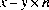whereis the mathematical integer closest to the exact mathematical value of the quotient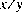; if two mathematical integers are equally close tothen n is the integer that is even. If the remainder is zero, its sign is the same as the sign of the first argument.

Special cases:

• If either argument is NaN, or the first argument is infinite, or the second argument is positive zero or negative zero, then the result is NaN.
• If the first argument is finite and the second argument is infinite, then the result is the same as the first argument.

20.11.15 `public static double ceil(double a)`

The result is the smallest (closest to negative infinity) `double` value that is not less than the argument and is equal to a mathematical integer.

Special cases:

• If the argument value is already equal to a mathematical integer, then the result is the same as the argument.
• If the argument is NaN or an infinity or positive zero or negative zero, then the result is the same as the argument.
• If the argument value is less than zero but greater than `-1.0`, then the result is negative zero.
Note that the value of `Math.ceil(x)` is exactly the value of `-Math.floor(-x)`.

20.11.16 `public static double floor(double a)`

The result is the largest (closest to positive infinity) `double` value that is not greater than the argument and is equal to a mathematical integer.

Special cases:

• If the argument value is already equal to a mathematical integer, then the result is the same as the argument.
• If the argument is NaN or an infinity or positive zero or negative zero, then the result is the same as the argument.

20.11.17 `public static double rint(double a)`

The result is the `double` value that is closest in value to the argument and is equal to a mathematical integer. If two `double` values that are mathematical integers are equally close to the value of the argument, the result is the integer value that is even.

Special cases:

• If the argument value is already equal to a mathematical integer, then the result is the same as the argument.
• If the argument is NaN or an infinity or positive zero or negative zero, then the result is the same as the argument.

20.11.18 `public static int round(float a)`

The result is rounded to an integer by adding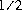, taking the floor of the result, and casting the result to type `int`.

In other words, the result is equal to the value of the expression:

```(int)Math.floor(a + 0.5f)
```
Special cases:

• If the argument is NaN, the result is `0`.
• If the argument is negative infinity, or indeed any value less than or equal to the value of `Integer.MIN_VALUE` (§20.7.1), the result is equal to the value of `Integer.MIN_VALUE`.
• If the argument is positive infinity, or indeed any value greater than or equal to the value of `Integer.MAX_VALUE` (§20.7.2), the result is equal to the value of `Integer.MAX_VALUE`.

20.11.19 `public static long round(double a)`

The result is rounded to an integer by adding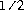, taking the floor of the result, and casting the result to type `long`.

In other words, the result is equal to the value of the expression:

```(long)Math.floor(a + 0.5d)
```
Special cases:

• If the argument is NaN, the result is `0`.
• If the argument is negative infinity, or indeed any value less than or equal to the value of `Long.MIN_VALUE` (§20.7.1), the result is equal to the value of `Long.MIN_VALUE`.
• If the argument is positive infinity, or indeed any value greater than or equal to the value of `Long.MAX_VALUE` (§20.7.2), the result is equal to the value of `Long.MAX_VALUE`.

20.11.20 `public static double random()`

The result is a double value with positive sign, greater than or equal to zero but less than `1.0`, chosen pseudorandomly with (approximately) uniform distribution from that range.

When this method is first called, it creates a single new pseudorandom-number generator, exactly as if by the expression

```new java.util.Random()
```
This new pseudorandom-number generator is used thereafter for all calls to this method and is used nowhere else.

This method is properly synchronized to allow correct use by more than one thread. However, if many threads need to generate pseudorandom numbers at a great rate, it may reduce contention for each thread to have its own pseudorandom number generator.

20.11.21 `public static int abs(int a)`

The result is the absolute value of the argument, if possible.

If the argument is not negative, the argument is returned.

If the argument is negative, the negation of the argument is returned. Note that if the argument is equal to the value of `Integer.MIN_VALUE` (§20.7.1), the most negative representable `int` value, the result will be that same negative value.

20.11.22 `public static long abs(long a)`

The result is the absolute value of the argument, if possible.

If the argument is not negative, the argument is returned.

If the argument is negative, the negation of the argument is returned. Note that if the argument is equal to the value of `Long.MIN_VALUE` (§20.8.1), the most negative representable `long` value, the result will be that same negative value.

20.11.23 `public static float abs(float a)`

The argument is returned with its sign changed to be positive.

Special cases:

• If the argument is positive zero or negative zero, the result is positive zero.
• If the argument is infinite, the result is positive infinity.
• If the argument is NaN, the result is NaN.
In other words, the result is equal to the value of the expression:

```Float.intBitsToFloat(0x7fffffff & Float.floatToIntBits(a))
```
[This specification for the method `abs` is scheduled for introduction in Java version 1.1. In previous versions of Java, `abs(-0.0f)` returns `-0.0f`, which is not correct.]

20.11.24 `public static double abs(double a)`

The argument is returned with its sign changed to be positive.

Special cases:

• If the argument is positive zero or negative zero, the result is positive zero.
• If the argument is infinite, the result is positive infinity.
• If the argument is NaN, the result is NaN.
In other words, the result is equal to the value of the expression:

```Double.longBitsToDouble((Double.doubleToLongBits(a)<<1)>>>1)
```
[This specification for the method `abs` is scheduled for introduction in Java version 1.1. In previous versions of Java, `abs(-0.0d)` returns `-0.0d`, which is not correct.]

20.11.25 `public static int min(int a, int b)`

The result is the smaller of the two arguments-that is, the one closer to the value of `Integer.MIN_VALUE` (§20.7.1). If the arguments have the same value, the result is that same value.

20.11.26 `public static long min(long a, long b)`

The result is the smaller of the two arguments-that is, the one closer to the value of `Long.MIN_VALUE` (§20.8.1). If the arguments have the same value, the result is that same value.

20.11.27 `public static float min(float a, float b)`

The result is the smaller of the two arguments-that is, the one closer to negative infinity. If the arguments have the same value, the result is that same value.

Special cases:

• If one argument is positive zero and the other is negative zero, the result is negative zero.
• If either argument is NaN, the result is NaN.
[This specification for the method `min` is scheduled for introduction in Java version 1.1. In previous versions of Java, `min(0.0f,` `-0.0f)` returns `0.0f`, which is not correct.]

20.11.28 `public static double min(double a, double b)`

The result is the smaller of the two arguments-that is, the one closer to negative infinity. If the arguments have the same value, the result is that same value.

Special cases:

• If one argument is positive zero and the other is negative zero, the result is negative zero.
• If either argument is NaN, the result is NaN.
[This specification for the method `min` is scheduled for introduction in Java version 1.1. In previous versions of Java, `min(0.0d,` `-0.0d)` returns `0.0d`, which is not correct.]

20.11.29 `public static int max(int a, int b)`

The result is the larger of the two arguments-that is, the one closer to the value of `Integer.MAX_VALUE` (§20.7.2). If the arguments have the same value, the result is that same value.

20.11.30 `public static long max(long a, long b)`

The result is the larger of the two arguments-that is, the one closer to the value of `Long.MAX_VALUE` (§20.8.2). If the arguments have the same value, the result is that same value.

20.11.31 `public static float max(float a, float b)`

The result is the larger of the two arguments-that is, the one closer to positive infinity. If the arguments have the same value, the result is that same value.

Special cases:

• If one argument is positive zero and the other is negative zero, the result is positive zero.
• If either argument is NaN, the result is NaN.
[This specification for the method `max` is scheduled for introduction in Java version 1.1. In previous versions of Java, `max(-0.0f,` `0.0f)` returns `-0.0f`, which is not correct.]

20.11.32 `public static double max(double a, double b)`

The result is the larger of the two arguments-that is, the one closer to positive infinity. If the arguments have the same value, the result is that same value.

Special cases:

• If one argument is positive zero and the other is negative zero, the result is positive zero.
• If either argument is NaN, the result is NaN.
[This specification for the method `max` is scheduled for introduction in Java version 1.1. In previous versions of Java, `max(-0.0d,` `0.0d)` returns `-0.0d`, which is not correct.].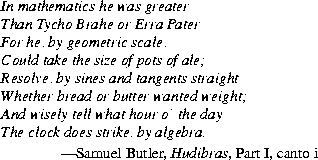Java Language Specification (HTML generated by Suzette Pelouch on February 24, 1998)